# Acute angles

 1 Introduction To Angles 2 What is an Acute Angle? 3 Definition of an Acute Angles 4 Examples of Acute Angles 5 Acute Angle Formula 6 Properties of Acute Triangles 7 Solved Examples on Acute Angles 8 Challenging Questions on Acute Angles 9 Practice Questions on Acute Angles 10 Important Notes on Acute Angles 11 Maths Olympiad Sample Papers 12 Frequently Asked Questions (FAQs)

We at Cuemath believe that Math is a life skill. Our Math Experts focus on the “Why” behind the “What.” Students can explore from a huge range of interactive worksheets, visuals, simulations, practice tests, and more to understand a concept in depth.

Book a FREE trial class today! and experience Cuemath’s LIVE Online Class with your child.

## Introduction To Angles

Do you know how an angle is formed?

When two rays meet at a vertex, an angle is formed.

An angle is represented by the symbol $$\angle$$ and is measured in degrees $$^\circ$$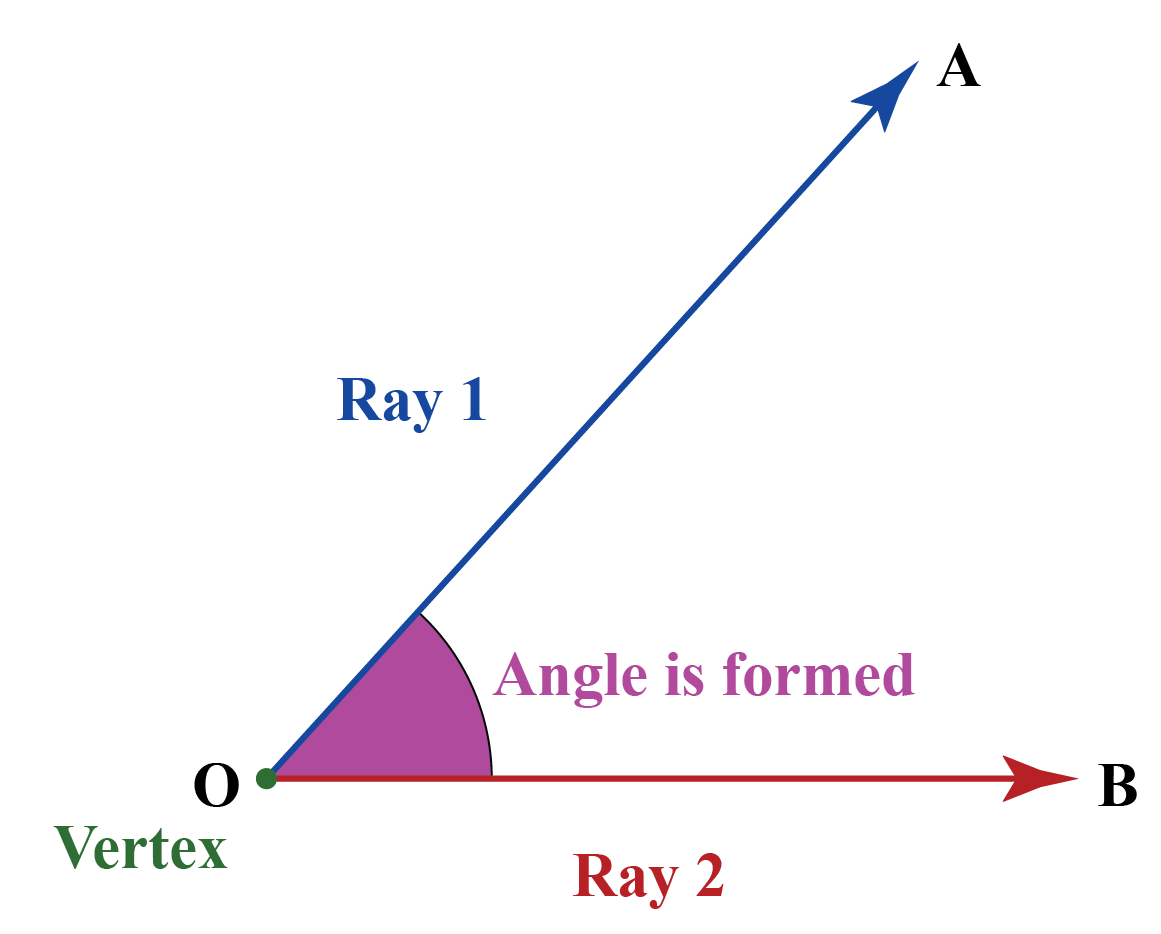There are different types of angles.

They can be acute, obtuse, reflex, straight, right and whole.

We will learn about acute angles in this topic.

## What is an Acute Angle?

An acute angle is an angle whose measure is greater than $${0^\circ}$$ and less than $${90^\circ}$$.

Here are a few examples of acute angles.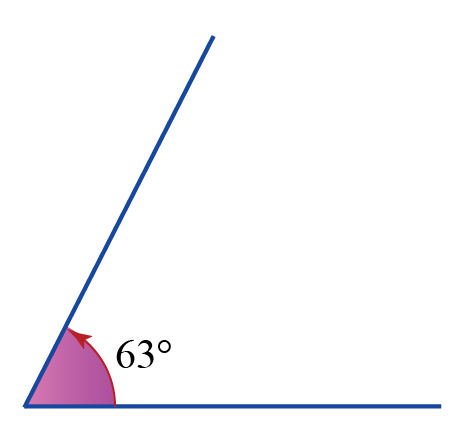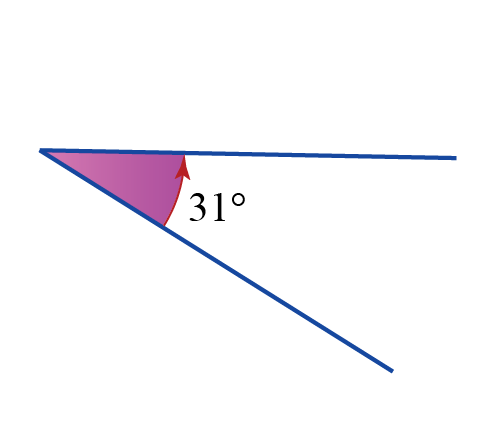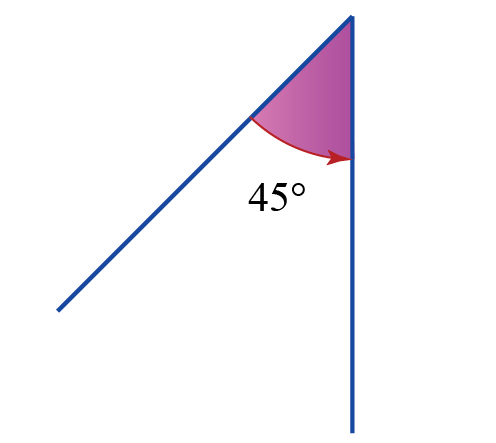## Definition of an Acute Angle

The definition of an acute angle states that an angle whose measure is greater than 0° and less than 90° is an acute angle.

Look at the simulation below.

Drag point B to see the acute angles that can be formed here.

## Examples of Acute Angles

We know that angles measuring greater than 0° and less than 90° are called acute angles in geometry.

Therefore, $$45^\circ$$, $$5^\circ$$, $$18^\circ$$, $$49^\circ$$, $$89^\circ$$ are all examples of acute angles.

Here are some real-life examples of acute angles.

• A  slice of watermelon
• An intersection of a crossroad
• Some instances of the angles formed between the hour's hand and the minute's hand of the clock
• Angle between the arms of a pair of scissors
• The beak of a bird when it is open
• An angle formed when a crocodile mouth is open

Look around and you will be observe other examples of acute angles.## Properties of Acute Triangles

An acute triangle has all its angles less than 90°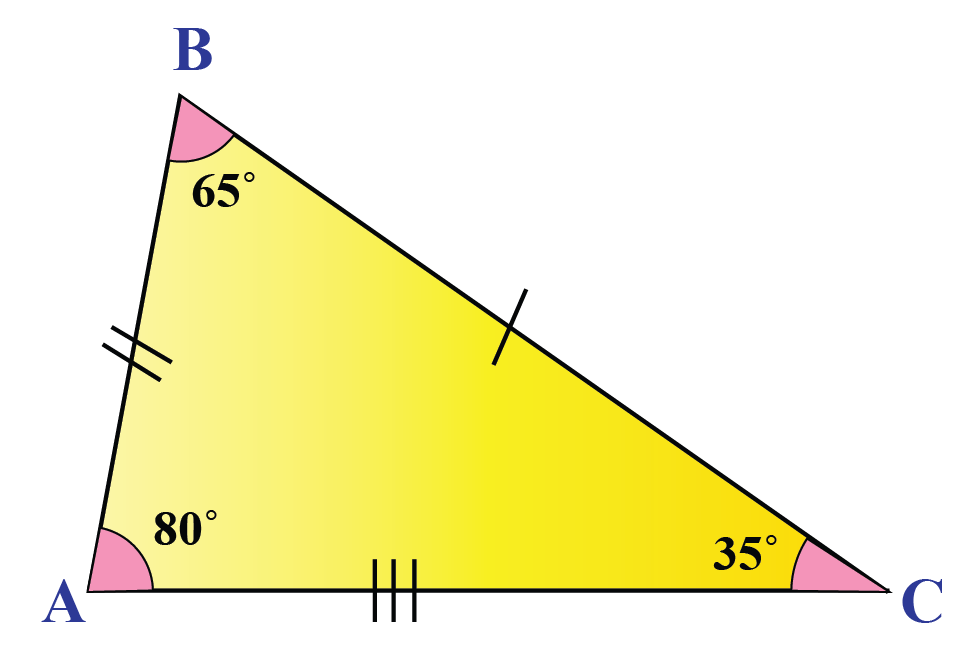When all three angles of a triangle are 60°, it forms a special triangle called an equilateral triangle.

Acute triangles can be classified as acute scalene triangles, acute isosceles triangles and equilateral triangles.

You can go through types of triangles for more information on these triangles.

## Acute Angle Formula

Just like the Pythagoras theorem for right triangles, we have a triangle inequality for acute triangles.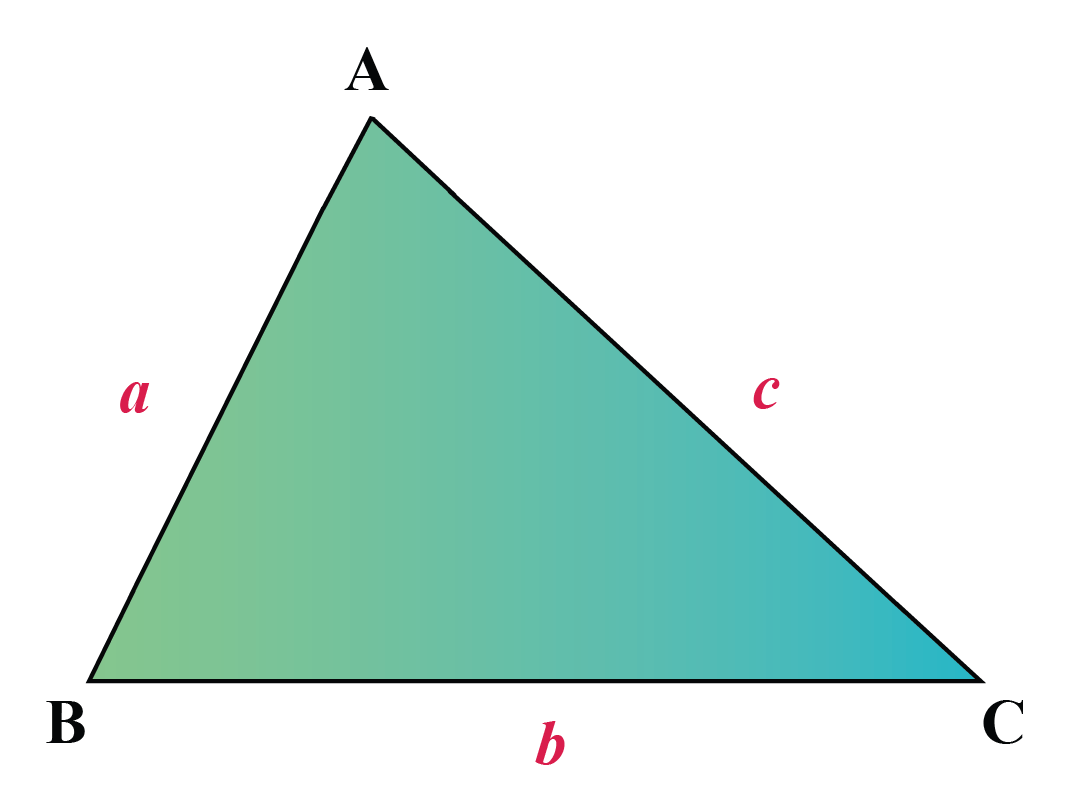It states that the sum of the squares of the two sides is greater than the square of the greatest side.

In $$\Delta ABC$$, the sides measure $$a,b,c$$ such that $$c$$ is the largest side, then:

 $$a^2 + b^2 > c^2$$

Conversely, in a triangle, if $$a^2 + b^2 >c^2$$, the triangle is an acute triangle.

Help your child score higher with Cuemath’s proprietary FREE Diagnostic Test. Get access to detailed reports, customised learning plans and a FREE counselling session. Attempt the test now.

## Solved Examples

 Example 1

Here is a small activity for you to try.

When you click on Generate New Angle, a random angle is generated.

Measure the angle formed using a protractor and identify if it is an acute angle.

Solution:

All the angles formed measure less than 90°

Hence, they are all acute angles.

 All the formed angles are acute angles.
 Example 2

From the following options, choose the angles which can be classified as acute angles.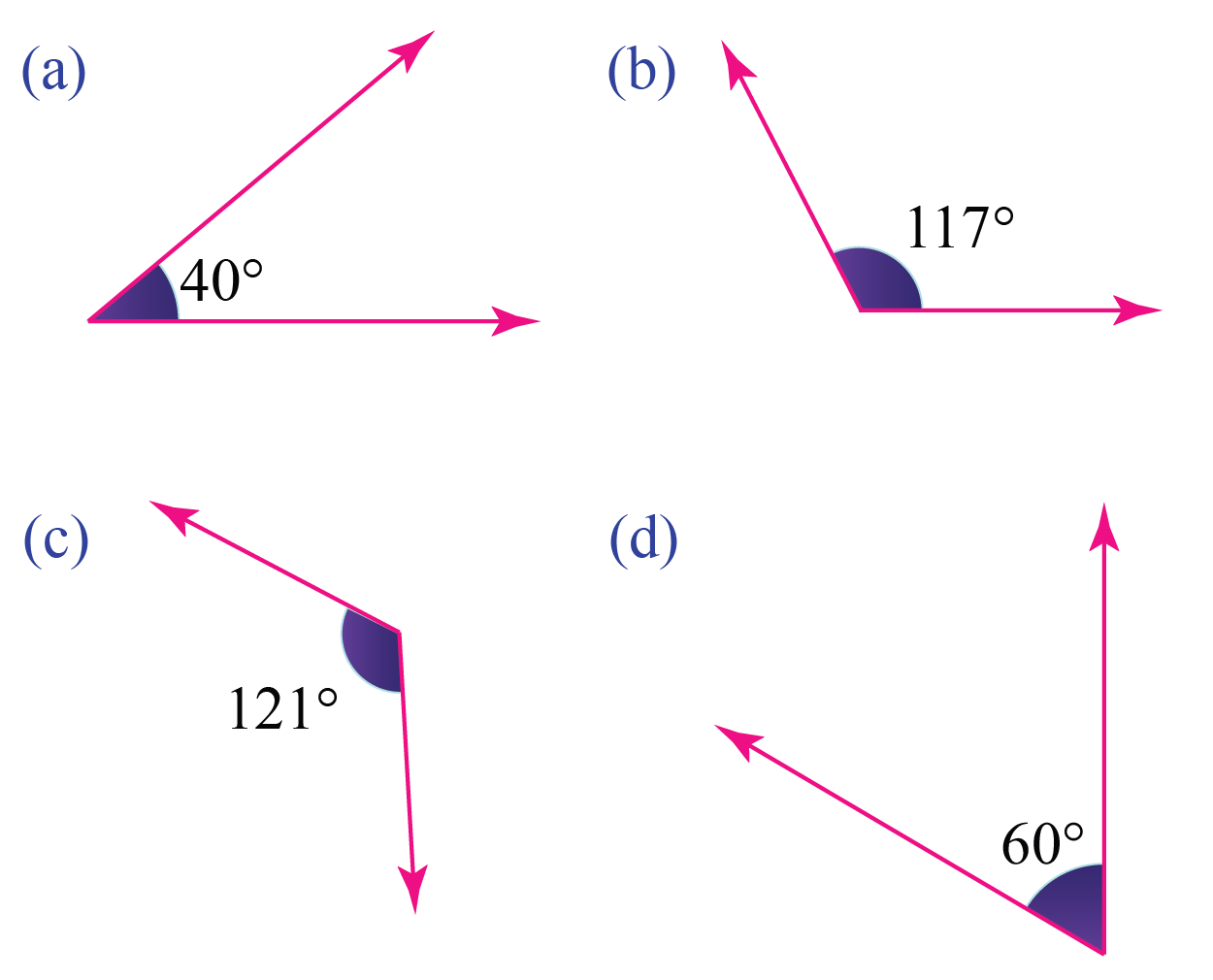Solution:

Option $$a$$ and option $$d$$ are less than $$90^\circ$$

Hence, they are acute angles.

 Option (a) and option (d) are acute angles.
 Example 3

At what time, in the clock shown below, an acute angle is formed?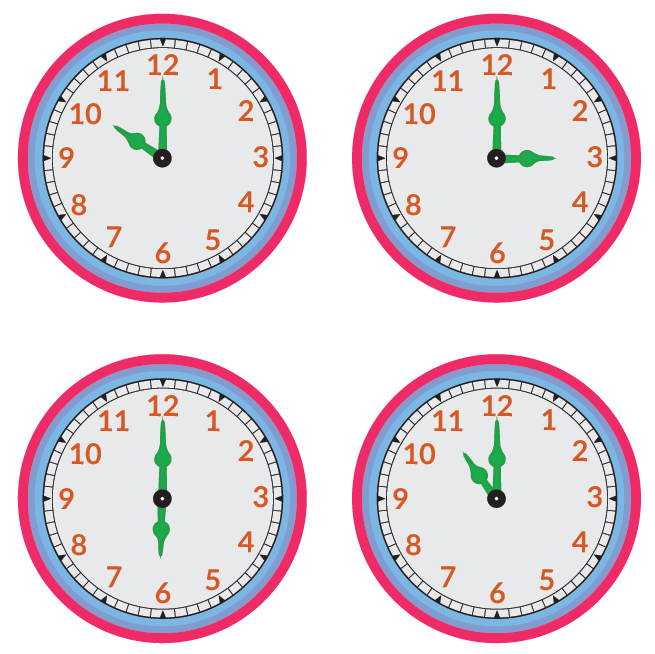Solution:

We can observe that at 10 o'clock and 11 o'clock, the angle formed between the hour hand and the minute hand is an acute angle.

 $$\therefore$$ An acute angle is formed at 11 o'clock and 10 o'clock.
 Example 4

Is the following triangle acute?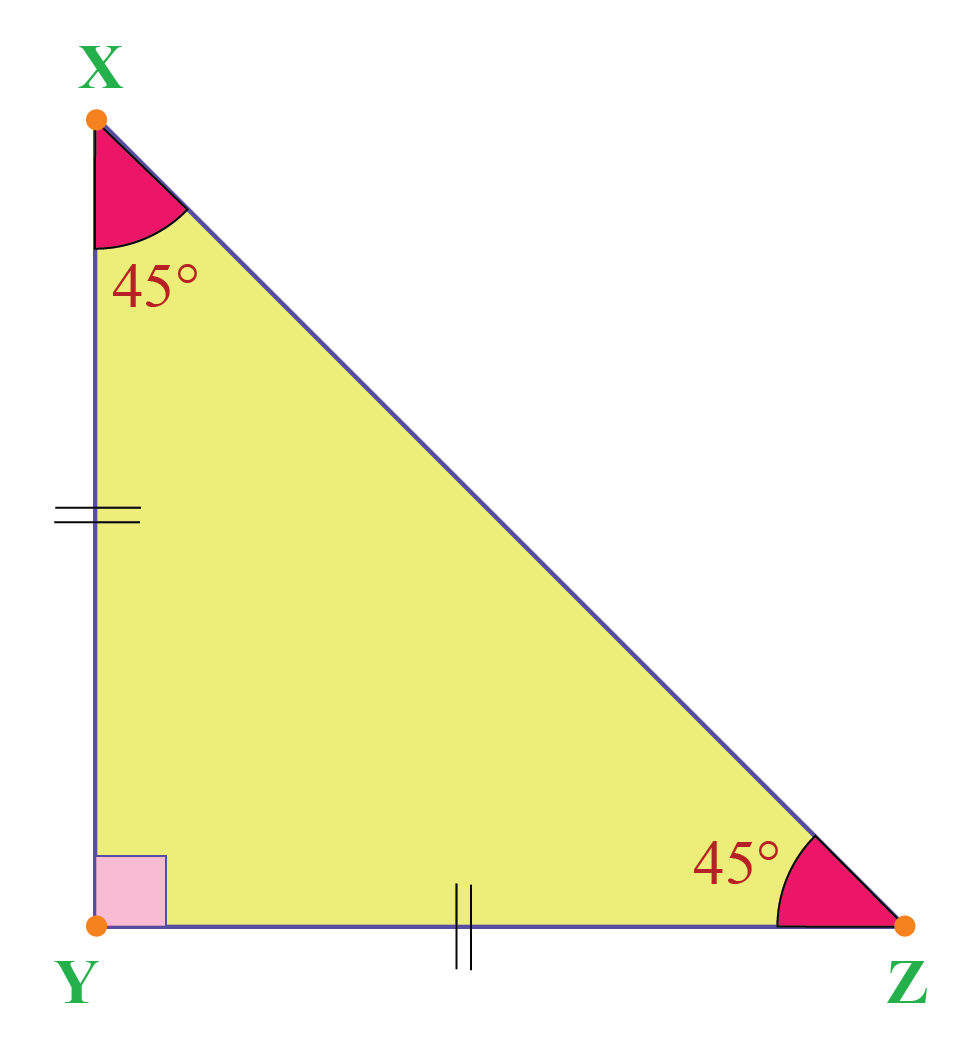Solution:

All the 3 angles should measure less than 90° in an acute triangle.

From the figure, we can see that two angles measure 45° each.

$$\angle Y$$ is a right angle.

Hence, this is not an acute triangle.

 The given triangle is not an acute triangle.
 Example 5

Can an acute triangle have sides that measure 4 cm, 5 cm, and 8 cm?

Solution:

In an acute triangle, the sum of the squares of the two sides should be greater than the square of the greatest side.

i.e   $$a^2 + b^2 >c^2$$ where c is the largest side.

Let a = 4 cm

b= 5 cm

c = 8 cm (largest side )

$$a^2 = 16$$

$$b^2 = 25$$

$$c^2 = 64$$

$$a^2 +b^2= 16 +25 = 41$$

Since  $$41 < 64$$

$$\implies a^2 + b^2$$  is less than $$c^2$$

Hence, the given measures do not form an acute triangle.

 4 cm, 5 cm and 8 cm cannot form an acute triangle.Challenging Questions
1. How many acute angles are there in the given images?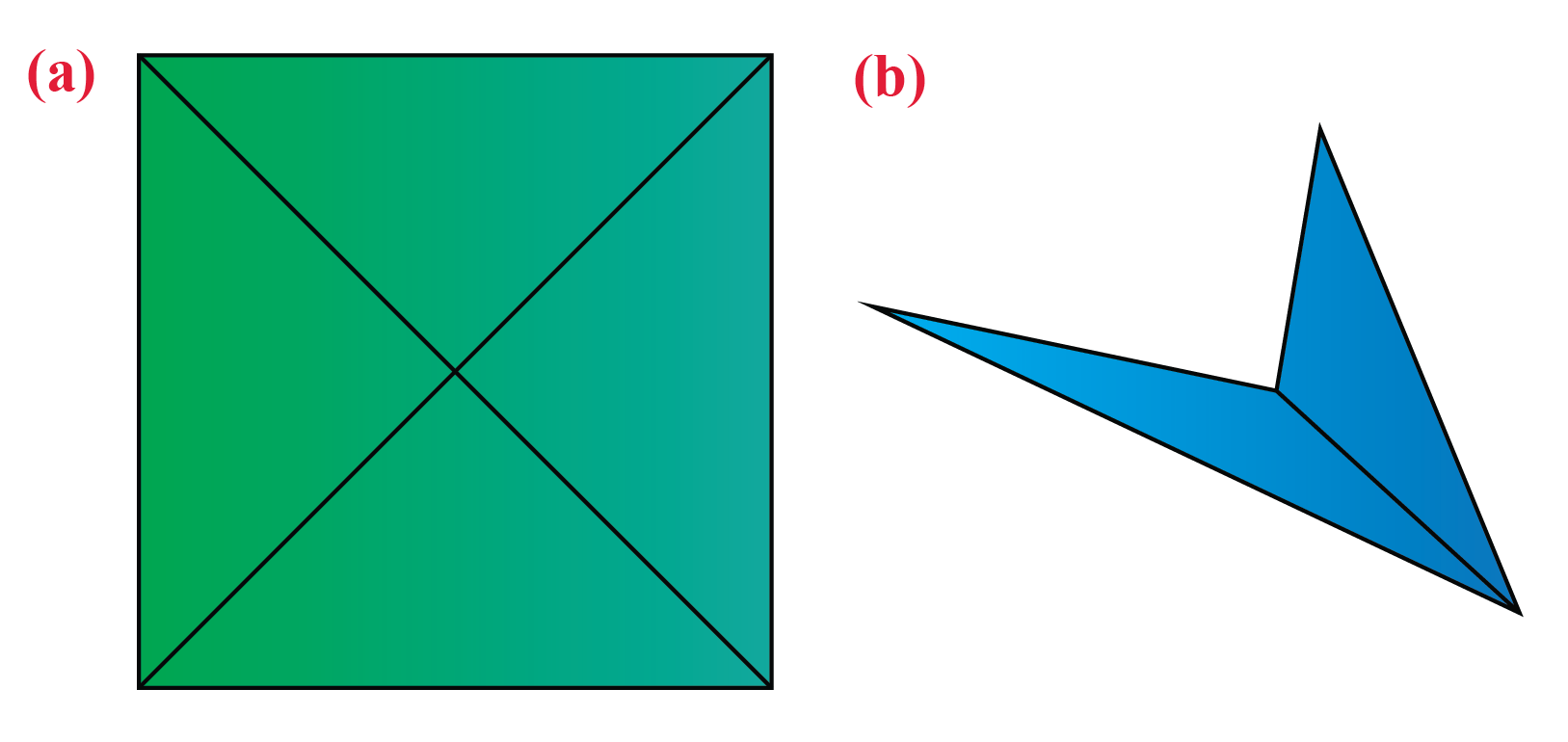CLUEless in Math? Check out how CUEMATH Teachers will explain Acute Angles to your kid using interactive simulations & worksheets so they never have to memorise anything in Math again!

Explore Cuemath Live, Interactive & Personalised Online Classes to make your kid a Math Expert. Book a FREE trial class today!

## Practice Questions

Here are a few activities for you to practice.

IMO (International Maths Olympiad) is a competitive exam in Mathematics conducted annually for school students. It encourages children to develop their math solving skills from a competition perspective.

## 1. What is the degree measure (number) of an acute angle?

An angle whose measure is greater than 0° and less than 90° is called an acute angle.

## 2. What is an acute angle triangle?

A triangle where all three vertex angles measure less than 90° is called an acute angle triangle.

## 3. What is an acute angle parallelogram?

In a parallelogram, the consecutive angles are supplementary and opposite angles are equal.

Hence, there can be two acute angles which are opposite to each other.

The other two angles are obtuse angles.

A rectangle/square is a special case of a parallelogram where all angles are right angles.

More Important Topics
Numbers
Algebra
Geometry
Measurement
Money
Data
Trigonometry
Calculus